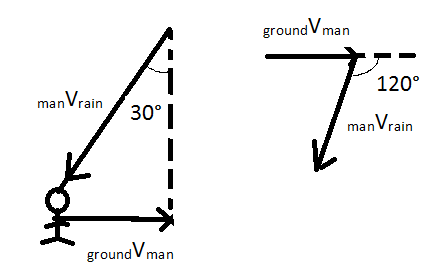# A person walking at 4m/s finds rain drops falling slantwise to his face with a speed of 4m/s at an angle of 30 degrees with the vertical. Show that the actual speed of the rain drops is 4m/s

9 years agoVelocity of rain wrt man= 4m/sat an angle 30º with vertical (given)

velocity of man wrt ground = 4m/s

from fig.

velocity of rain wrt man + velocity of man wrt ground = velocity of rain wrt ground (triangle law of vector addition)

so, velocity of rain wrt ground = √(4)2 + (4)2 + 2(4)(4)cos 120

= √ 16+16+32(-0.5)

=√32-16

=√16 = 4m/s

hence proved!

3 years ago
Vrm=4 m/s
Theta=30°
Vmg= 4 m/s
According to triangle law of vectors,
Velocity of man w.r.t ground, Vrg
=Vrm + Vmg
=Vrg
=√4*4 + 4*4 + 2*4*4cos120
=√32 - 16
=√16
=4
Hence proved